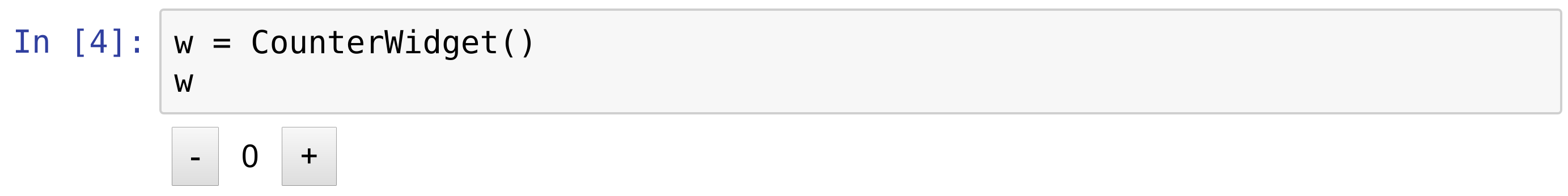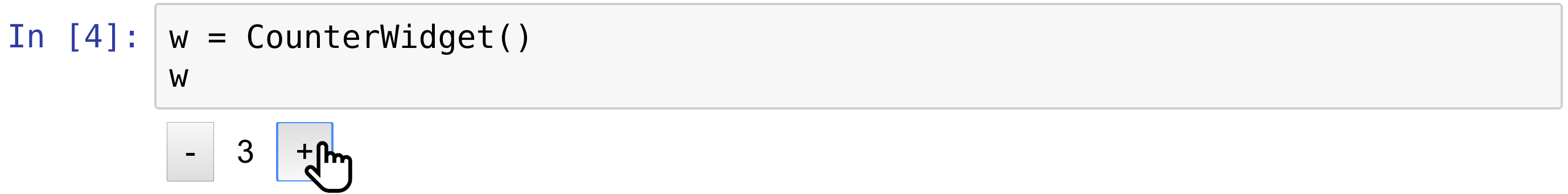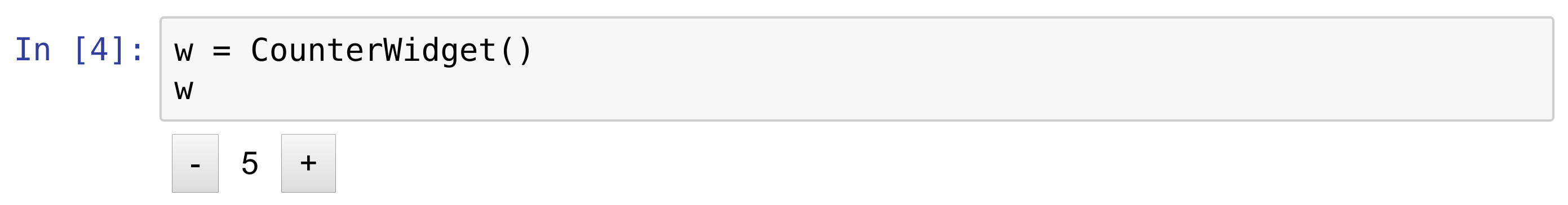This is one of the 100+ free recipes of the IPython Cookbook, Second Edition, by Cyrille Rossant, a guide to numerical computing and data science in the Jupyter Notebook. The ebook and printed book are available for purchase at Packt Publishing.

▶  Text on GitHub with a CC-BY-NC-ND license
▶  Code on GitHub with a MIT license

The ipywidgets packages provides many built-in control widgets to interact with code and data in the Jupyter Notebook. In this recipe, we show how to build a custom interactive widget from scratch, using Python on the kernel side, and HTML/JavaScript on the client side (frontend). The widget just displays two buttons to increase and decrease a number. The number can be accessed and updated either from the kernel (Python code) or the client (browser).

## How to do it...

1.  Let's import the packages:

```import ipywidgets as widgets
from traitlets import Unicode, Int, validate
```

2.  We create a `CounterWidget` class deriving from `DOMWidget`:

```class CounterWidget(widgets.DOMWidget):
_view_name = Unicode('CounterView').tag(sync=True)
_view_module = Unicode('counter').tag(sync=True)
value = Int(0).tag(sync=True)
```

This class represents the Python part of the widget. The `_view_name` and `_view_module` attributes refer to the name and module of the JavaScript part. We use the traitlets package to specify the type of the variables. The `value` attribute is the counter value, an integer initialized at 0. All of these attributes' values are synchronized between Python and JavaScript, hence the `sync=True` option.

3.  We now turn to the JavaScript side of the widget. We can write the code directly in the notebook using the `%%javascript` cell magic. The widgets framework relies on several JavaScript libraries: jQuery (represented as the `\$` variable), require.js (modules and dependencies), and Backbone.js (a model view controller framework):

```%%javascript
// We make sure the `counter` module is defined
// only once.
require.undef('counter');

// We define the `counter` module depending on the
// Jupyter widgets framework.
define('counter', ["@jupyter-widgets/base"],
function(widgets) {

// We create the CounterView frontend class,
// deriving from DOMWidgetView.
var CounterView = widgets.DOMWidgetView.extend({

// This method creates the HTML widget.
render: function() {
// The value_changed() method should be
// called when the model's value changes
// on the kernel side.
this.value_changed();
this.model.on('change:value',
this.value_changed, this);

var model = this.model;
var that = this;

// We create the plus and minus buttons.
this.bm = \$('<button/>')
.text('-')
.click(function() {
// When the button is clicked,
// the model's value is updated.
var x = model.get('value');
model.set('value', x - 1);
that.touch();
});

this.bp = \$('<button/>')
.text('+')
.click(function() {
var x = model.get('value');
model.set('value', x + 1);
that.touch();
});

// This element displays the current
// value of the counter.
this.span = \$('<span />')
.text('0')
.css({marginLeft: '10px',
marginRight: '10px'});

// this.el represents the widget's DOM
// element. We add the minus button,
// the span element, and the plus button.
\$(this.el)
.append(this.bm)
.append(this.span)
.append(this.bp);
},

value_changed: function() {
// Update the displayed number when the
// counter's value changes.
var x = this.model.get('value');
\$(\$(this.el).children()).text(x);
},
});

return {
CounterView : CounterView
};
});
```

4.  Let's display the widget:

```w = CounterWidget()
w
```5.  Pressing the buttons updates the value immediately.6.  The counter's value is automatically updated on the kernel side:

```print(w.value)
```
```4
```

7.  Conversely, we can update the value from Python, and it is updated in the frontend:

```w.value = 5
```## There's more...

Here are a few references: